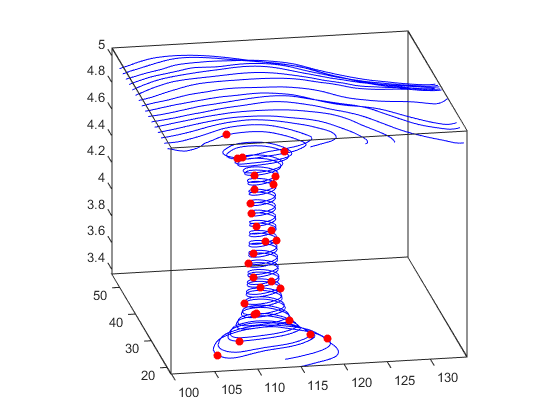## 创建流粒子动画

### 随时间变化的抛射体路径

`$z\left(t\right)={v}_{z}t+\frac{a{t}^{2}}{2}$`

```vz = 10; % velocity constant a = -32; % acceleration constant t = 0:.1:1; z = vz*t + 1/2*a*t.^2;```

```vx = 2; x = vx*t; vy = 3; y = vy*t;```

```u = gradient(x); v = gradient(y); w = gradient(z); scale = 0; figure quiver3(x,y,z,u,v,w,scale) view([70,18])```### 1.指定数据范围的起点

```load wind [sx sy sz] = meshgrid(100,20:2:50,5);```

### 2.创建流线以指示粒子路径

```verts = stream3(x,y,z,u,v,w,sx,sy,sz); sl = streamline(verts);```

### 3.定义视图

• 选择的视点 (`view`) 既能显示包含大部分流线的平面，又能显示螺旋线。

• 将数据的纵横比 (`daspect`) 设为 `[2 2 0.125]` 可在 z 方向提供更高的分辨率，使螺旋线中的流粒子更容易看清。

• 将坐标区范围设置为与数据范围匹配 (`axis`)，然后绘制轴框 (`box`)。

```view(-10.5,18) daspect([2 2 0.125]) axis tight; set(gca,'BoxStyle','full','Box','on') ```

### 4.计算流粒子顶点

`streamparticles` 函数设置以下属性：

• `Animate` 设置为 `10`，使动画运行 10 次。

• `ParticleAlignment` 设置为 `on`，以便同时开始所有粒子跟踪。

• `MarkerEdgeColor` 设置为 `none`，以便只绘制圆形标记的面。如果不绘制标记的边，动画通常会运行得更快。

• `MarkerFaceColor` 设置为 `red`

• `Marker` 设置为 `o`，以绘制圆形标记。您也可以使用其他线标记。

```iverts = interpstreamspeed(x,y,z,u,v,w,verts,0.01); set(gca,'SortMethod','childorder'); streamparticles(iverts,15,... 'Animate',10,... 'ParticleAlignment','on',... 'MarkerEdgeColor','none',... 'MarkerFaceColor','red',... 'Marker','o');```